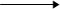Visitors Online: 77 | Saturday 24th August 2019CBSE Guess > Papers > Important Questions > Class XII > 2013 > Chemistry > Nernst Equation (Numerical) By Mr. R. Srinivas Vasudevamurthy CBSE CLASS XII
Nernst Equation (Numerical) 5. Calculate the potential of hydrogen electrode in contact with a solution of pH = 10. 6. At what pH of HCl solution will the standard hydrogen electrode will have a potential of - 0.118V ? 7. Calculate the cell potential of Cr/Cr3+|| Fe2 / Fe.(0.1M) (0.1M) Given E0Cr3+/Cr = - 0.74V E0 Fe2+/Fe = - 0.44V. 8. Calculate the cell potential at 298K Zn + Sn4+Zn2+  + Sn2+ (1.5M)                         (0.5M)  (2M)  Given E0Zn2+/Zn = - 0.76V E0Sn4+/Sn2+ = 0.13V 9. Calculate the equilibrium constant and work done by the cellNi + Cu2+ Ni2+ +Cu Given E0Ni2+ / Ni = - 0.25V E0 Cu2+/Cu = 0.34 V 10 . Find the emf of the cell Pb/Pb2+(0.001M)|| Pt, Cl2 (1.5 atm) / 2Cl-(1M) E0Pb2+/ Pb = - 0.13V  E0 Cl2/ 2 Cl- = 1.36V 11. Find the potential of the electrode Mg2+/ Mg Mg2+ + 2e-Mg E0 Mg2+/ Mg = - 2.36 V 12. An electrochemical cell is set up by dipping Cu in 0.1M CuSO4(aq) and Ag in 0.1M AgNO3(aq) Write reaction taking place at each electrode and overall reaction. Determine the potential of the cell at 298K.   Submitted By : Mr. R. Srinivas Vasudevamurthy Email: vasudm@yahoo.co.uk